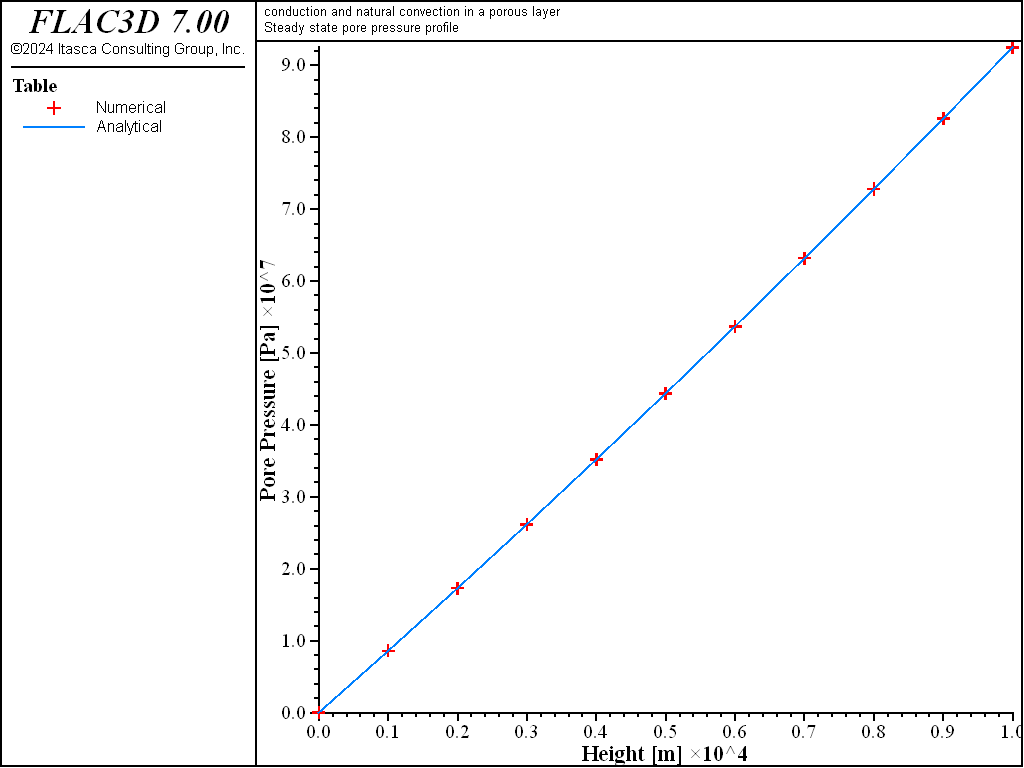# Steady-State Convection in a Saturated Porous Medium Heated from Above

Note

To view this project in FLAC3D, use the menu command Help ► Examples…. Choose “Thermal/NaturalAdvection” and select “NaturalAdvection.prj” to load. The project’s main data files are shown at the end of this example.

This example shows the effect of temperature-dependent density on the pore-pressure distribution for heating applied from above a saturated porous layer of large lateral extent. The thickness of the layer is $$b$$. The temperature is fixed at $$T_1$$ at the base of the layer and at $$T_0 > T_1$$ at the top. The boundaries are impermeable to fluid flow; the problem is one-dimensional. For zero specific discharge, the steady-state temperature solution is

(1)$T = {{T_1 - T_0} \over {b}} x + T_0$

where $$x$$ is measured down, from the top of the layer. Assuming no fluid flow, we have

(2)${dp \over dx} = \rho g$

Using $$\rho = \rho_0[1 - \beta (T - T_r)]$$, where $$T_r$$ is a reference temperature and $$T$$ is given by the temperature solution (Equation (1)), integration of this equation gives

(3)$p = \rho_0 gx - \rho_0 g \beta [(T_0 - T_r)x + {{T_1 - T_0} \over b} {x^2 \over 2}] + p_0$

where $$p_0$$ is a reference pressure.

To simulate the problem with FLAC3D, we first establish the temperature field and cycle in flow mode until the steady-state pore pressure solution is reached. For the case treated, the relative pore-pressure error calculated at the end of the simulation is negligible (less than 10-5%; see Figure 1).Figure 1: Comparison of numerical (symbols) and analytical (solid lines) pore pressure profiles at steady state for a porous saturated layer heated from above.

Data File

model new
model title  'conduction and natural convection in a porous layer'
fish automatic-create off
model config fluid thermal
program call "fishFunctions"
[setup]
; --- geometry ---
zone create brick size 5 5 10 point 0 (-5e3,-5e3,-5e3) ...
point 1 (5e3,-5e3,-5e3)  ...
point 2 (-5e3,5e3,-5e3)  ...
point 3 (-5e3,-5e3,5e3)
; --- thermal and fluid models ---
zone fluid cmodel assign isotropic
; --- properties ---
;    (mechanical)
zone initialize density 2000
;    (thermal)
zone thermal property conductivity=[_condu] specific-heat=[_mspec]
zone thermal property conductivity-fluid=[_fcond] specific-heat-fluid=[_fspec]
zone thermal property expansion-fluid=[_fthex] expansion=[_thexp]
zone thermal property temperature-reference=[_T1]
;    (fluid)
zone fluid property porosity=[_poros] permeability=[_mobil]
zone fluid property biot=1 undrained-thermal-coefficient=0
zone fluid biot off
zone gridpoint initialize fluid-modulus=2e5
zone gridpoint initialize fluid-tension=-1e10
zone initialize fluid-density=[_fdens]
; --- initial conditions ---
; --- boundary conditions ---
zone face apply temperature [_T1] range position-z -5e3
zone face apply temperature [_T0] range position-z  5e3
; --- settings ---
model gravity 0 0 -10.0
model mechanical active off
; --- conduction solution ---
zone initialize fluid-density=0.0
model fluid active off
model thermal active on
model step 500
zone initialize fluid-density=[_fdens]
model thermal active off
model fluid active on
model step 500
[ref_pp]
fish list [err_pp] [err_t]
[_makeTables]